## Figures index

#### Miroslav Pástor, Jozef Bocko, Tomáš Kula, Cosmin-Stefan Roszkos

American Journal of Mechanical Engineering. 2016, 4(7), 357-362 doi:10.12691/ajme-4-7-22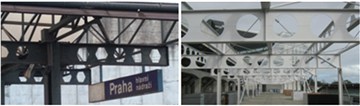• Figure 1. View of the roof structure formed from the castellated beams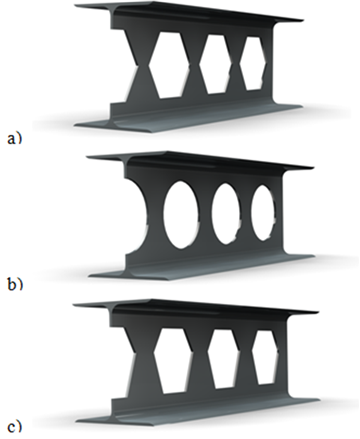• Figure 2. Typology of castellated and cellular beams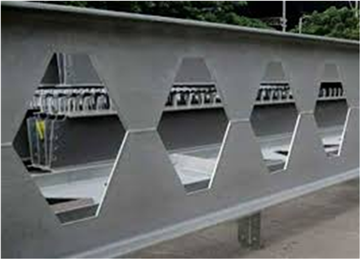• Figure 3. Bridge construction created by castellated beams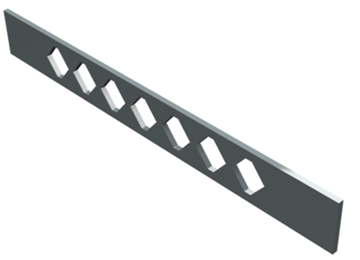• Figure 4. CAD beam model with holes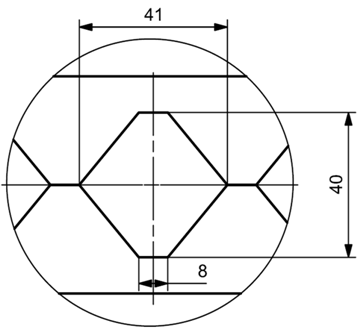• Figure 5. Shape and dimensions of hole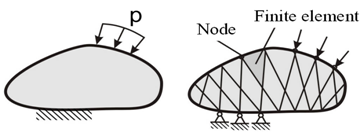• Figure 6. Solution of boundary problem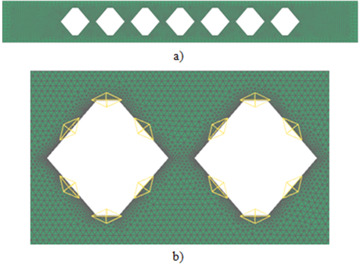• Figure 7. Mesh of finite elements a) whole model, b) detail of mesh around concentrators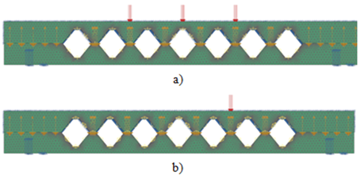• Figure 8. Defined boundary conditions for load condition a) Variant A, C, b) Variant B, D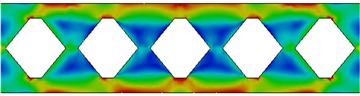• Figure 9. Field of equal difference of principal stresses σ1 - σ3 around the concentrator – variant A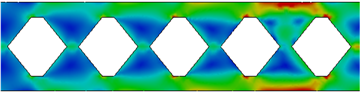• Figure 10. Field of equal difference of principal stresses σ1 - σ3 around the concentrator – variant B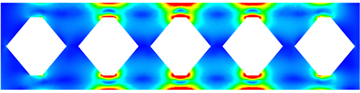• Figure 11. Field of equal difference of principal stresses σ1 - σ3 around the concentrator – variant C• Figure 12. Field of equal difference of principal stresses σ1 - σ3 around the concentrator – variant D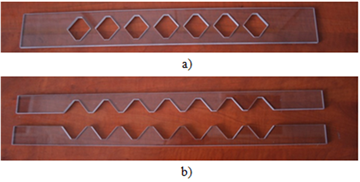• Figure 13. The models of the castellated beam produced from optically sensitive material PSM-1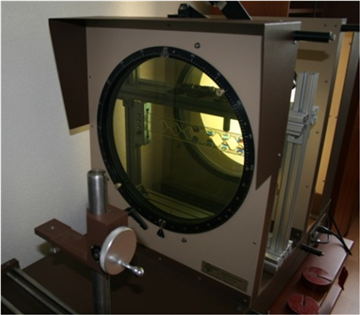• Figure 14. Measuring stand in the polariscope workspace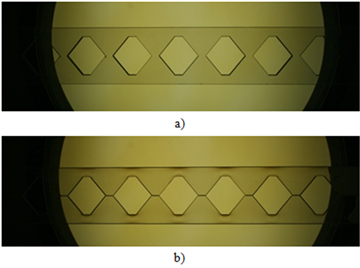• Figure 15. Castellated beams without loads a) connected parts, b) not connected parts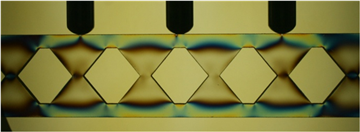• Figure 16. Field of isochromatics around analyzed concentrators – variant A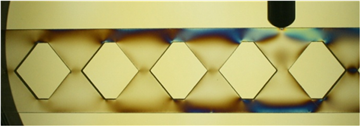• Figure 17. Field of isochromatics around analyzed concentrators – variant B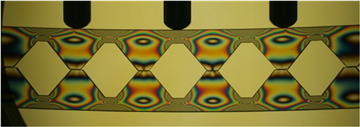• Figure 18. Field of isochromatics around analyzed concentrators – variant C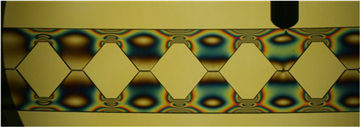• Figure 19. Field of isochromatics around analyzed concentrators – variant D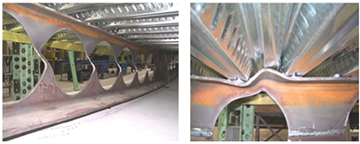• Figure 20. Collapsed cellular beam